Next: Electromagnetic Field Tensor Up: Relativity and Electromagnetism Previous: Retarded Potentials

# Tensors and Pseudo-Tensors

The totally antisymmetric fourth rank tensor is defined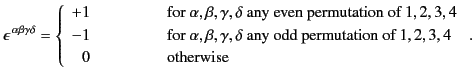(1754)

The components of this tensor are invariant under a general Lorentz transformation, because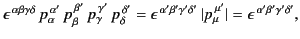(1755)

where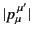denotes the determinant of the transformation matrix, or the Jacobian of the transformation, which we have already established is unity for a general Lorentz transformation. We can also define a totally antisymmetric third rank tensor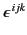which stands in the same relation to 3-space as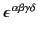does to space-time. It is easily demonstrated that the elements ofare invariant under a general translation or rotation of the coordinate axes. The totally antisymmetric third rank tensor is used to define the cross product of two 3-vectors,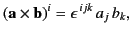(1756)

and the curl of a 3-vector field,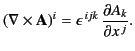(1757)

The following two rules are often useful in deriving vector identities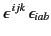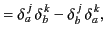(1758)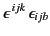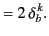(1759)

Up to now, we have restricted ourselves to three basic types of coordinate transformation: namely, translations, rotations, and standard Lorentz transformations. An arbitrary combination of these three transformations constitutes a general Lorentz transformation. Let us now extend our investigations to include a fourth type of transformation known as a parity inversion: that is,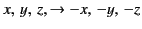. A reflection is a combination of a parity inversion and a rotation. As is easily demonstrated, the Jacobian of a parity inversion is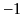, unlike a translation, rotation, or standard Lorentz transformation, which all possess Jacobians of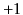.

The prototype of all 3-vectors is the difference in coordinates between two points in space,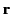. Likewise, the prototype of all 4-vectors is the difference in coordinates between two events in space-time,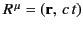. It is not difficult to appreciate that both of these objects are invariant under a parity transformation (in the sense that they correspond to the same geometric object before and after the transformation). It follows that any 3- or 4-tensor which is directly related toand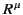, respectively, is also invariant under a parity inversion. Such tensors include the distance between two points in 3-space, the interval between two points in space-time, 3-velocity, 3-acceleration, 4-velocity, 4-acceleration, and the metric tensor. Tensors that exhibit tensor behavior under translations, rotations, special Lorentz transformations, and are invariant under parity inversions, are termed proper tensors, or sometimes polar tensors. Because electric charge is clearly invariant under such transformations (i.e., it is a proper scalar), it follows that 3-current and 4-current are proper vectors. It is also clear from Equation (1739) that the scalar potential, the vector potential, and the potential 4-vector, are proper tensors.

It follows from Equation (1756) that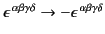under a parity inversion. Tensors such as this, which exhibit tensor behavior under translations, rotations, and special Lorentz transformations, but are not invariant under parity inversions (in the sense that they correspond to different geometric objects before and after the transformation), are called pseudo-tensors, or sometimes axial tensors. Equations (1758) and (1759) imply that the cross product of two proper vectors is a pseudo-vector, and the curl of a proper vector field is a pseudo-vector field.

One particularly simple way of performing a parity transformation is to exchange positive and negative numbers on the three Cartesian axes. A proper vector is unaffected by such a procedure (i.e., its magnitude and direction are the same before and after). On the other hand, a pseudo-vector ends up pointing in the opposite direction after the axes are renumbered.

What is the fundamental difference between proper tensors and pseudo-tensors? The answer is that all pseudo-tensors are defined according to a handedness convention. For instance, the cross product between two vectors is conventionally defined according to a right-hand rule. The only reason for this is that the majority of human beings are right-handed. Presumably, if the opposite were true then cross products, et cetera, would be defined according to a left-hand rule, and would, therefore, take minus their conventional values. The totally antisymmetric tensor is the prototype pseudo-tensor, and is, of course, conventionally defined with respect to a right-handed spatial coordinate system. A parity inversion converts left into right, and vice versa, and, thereby, effectively swaps left- and right-handed conventions.

The use of conventions in physics is perfectly acceptable provided that we recognize that they are conventions, and are consistent in our use of them. It follows that laws of physics cannot contain mixtures of tensors and pseudo-tensors, otherwise they would depend our choice of handedness convention.Let us now consider electric and magnetic fields. We know that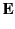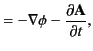(1760)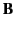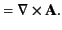(1761)

We have already seen that the scalar and the vector potential are proper scalars and vectors, respectively. It follows that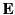is a proper vector, but that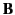is a pseudo-vector (because it is the curl of a proper vector). In order to fully appreciate the difference between electric and magnetic fields, let us consider a thought experiment first proposed by Richard Feynman. Suppose that we are in radio contact with a race of aliens, and are trying to explain to them our system of physics. Suppose, further, that the aliens live sufficiently far away from us that there are no common objects that we can both see. The question is this: could we unambiguously explain to these aliens our concepts of electric and magnetic fields? We could certainly explain electric and magnetic lines of force. The former are the paths of charged particles (assuming that the particles are subject only to electric fields), and the latter can be mapped out using small test magnets. We could also explain how we put arrows on electric lines of force to convert them into electric field-lines: the arrows run from positive charges (i.e., charges with the same sign as atomic nuclei) to negative charges. This explanation is unambiguous provided that our aliens live in a matter (rather than an anti-matter) dominated part of the universe. But, could we explain how we put arrows on magnetic lines of force in order to convert them into magnetic field-lines? The answer is no. By definition, magnetic field-lines emerge from the north poles of permanent magnets and converge on the corresponding south poles. The definition of the north pole of a magnet is simply that it possesses the same magnetic polarity as the south (geographic) pole of the Earth. This is obviously a convention. In fact, we could redefine magnetic field-lines to run from the south poles to the north poles of magnets without significantly altering our laws of physics (we would just have to replaceby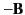in all our equations). In a parity inverted universe, a north pole becomes a south pole, and vice versa, so it is hardly surprising that.Next: Electromagnetic Field Tensor Up: Relativity and Electromagnetism Previous: Retarded Potentials
Richard Fitzpatrick 2014-06-27